# 序列推荐(transformer)

## Attention演进(RNN&LSTM&GRU&Seq2Seq + Attention机制)

### LSTM

LSTM是RNN的一种变体，RNN由于梯度消失只有短期记忆，而LSTM网络通过精妙的门控制，一定程度上缓解了梯度消失的问题。
LSTM得神经网络模块具有不同的结构，LSTM包含遗忘门、输入门和输出门，增加了非线性的相互作用。整体结构如图所示：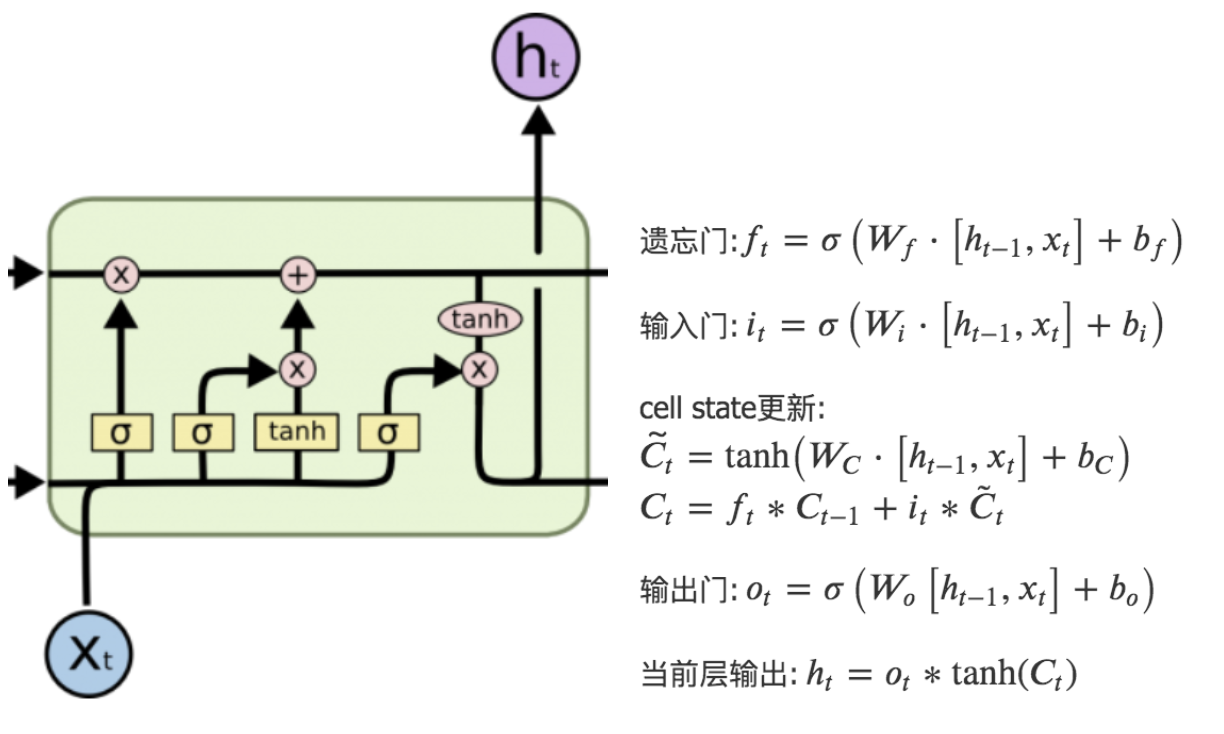cell state更新:
$\tilde{C_t}=\tanh \left(W_{C} \cdot\left[h_{t-1}, x_{t}\right]+b_{C}\right)$
$C_{t}=f_{t} * C_{t-1}+i_{t} * \tilde{C}_{t}$

### GRU

GRU是LSTM的一种变体，也是为了解决梯度消失（即长期记忆问题）而提出来的。相较于LSTM，GRU的网络结构更加简单，且效果很好。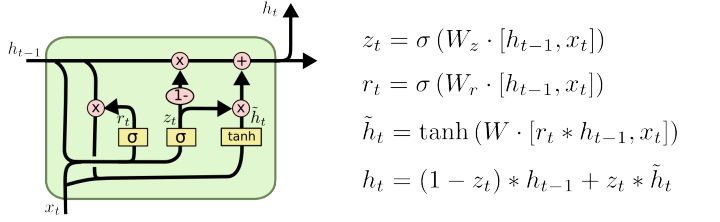### Seq2Seq + Attention机制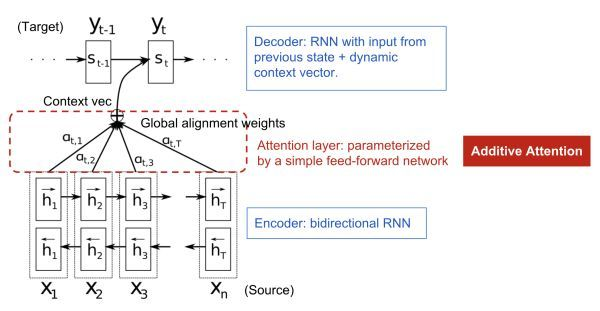## Attention机制(self-attention)

Attention其实就是计算一种相关程度；Attention通常可以进行如下描述，表示为将query(Q)和key-value pairs映射到输出上，其中query、每个key、每个value都是向量，输出是V中所有values的加权，其中权重是由Query和每个key计算出来的，计算方法分为三步：
1)计算比较Q和K的相似度，用f来表示：

$f\left(Q, K_{i}\right) , i=1,2,3$

2)将得到的相似度进行softmax归一化：

$\alpha_{i}=\frac{e^{f\left(Q, K_{i}\right)}}{\sum_{j=1}^{m} e^{f\left(Q, K_{i}\right)}}, i=1,2,3, . .$

3)针对计算出来的权重，对所有的values进行加权求和，得到Attention向量：

$\sum_{i=1}^m a_i V_i$

### 变种之Memory-based Attention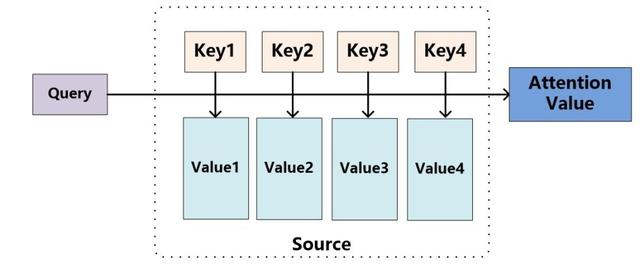Q&A 任务中，k 是 question，v 是 answer，q 是新来的 question，看看历史 memory 中 q 和哪个 k 更相似，然后依葫芦画瓢，根据相似 k 对应的 v，合成当前 question 的 answer。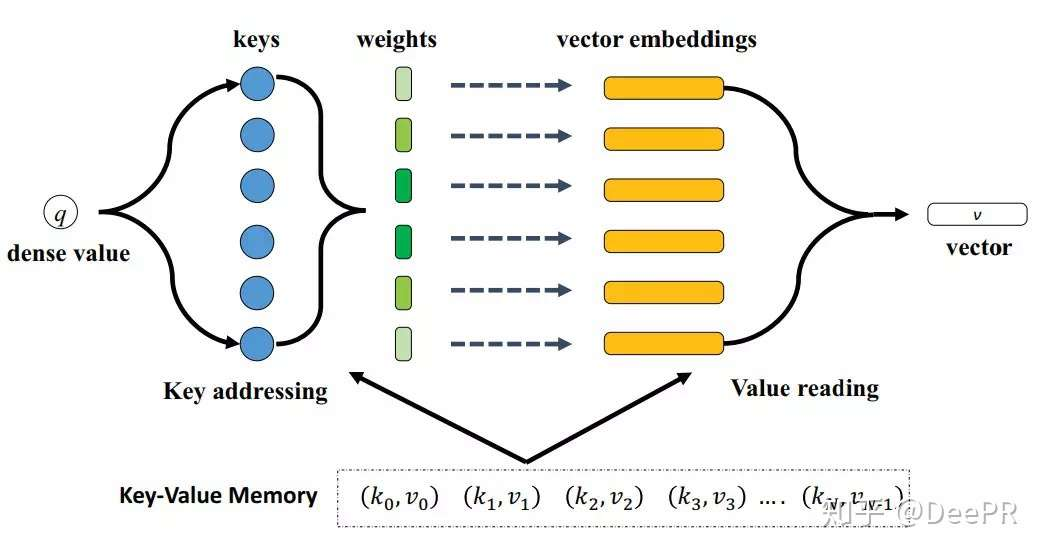### 变种之Soft/Hard Attention

hard attention 是一个随机采样，采样集合是输入向量的集合，采样的概率分布即attention weight。因此，hard attention 的输出是某一个特定的输入向量。
soft attention 是一个带权求和的过程，求和集合是输入向量的集合，对应权重是 相似度function 产出的 attention weight。

soft attention 是更常用的,下面讨论相似度function 【3】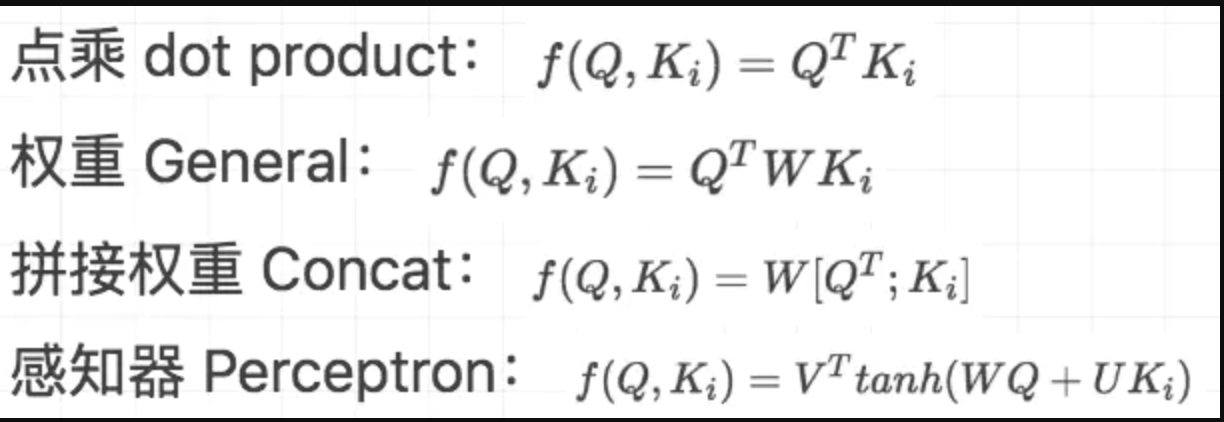### 变种之self-attention

#### Scaled Dot-Product Attention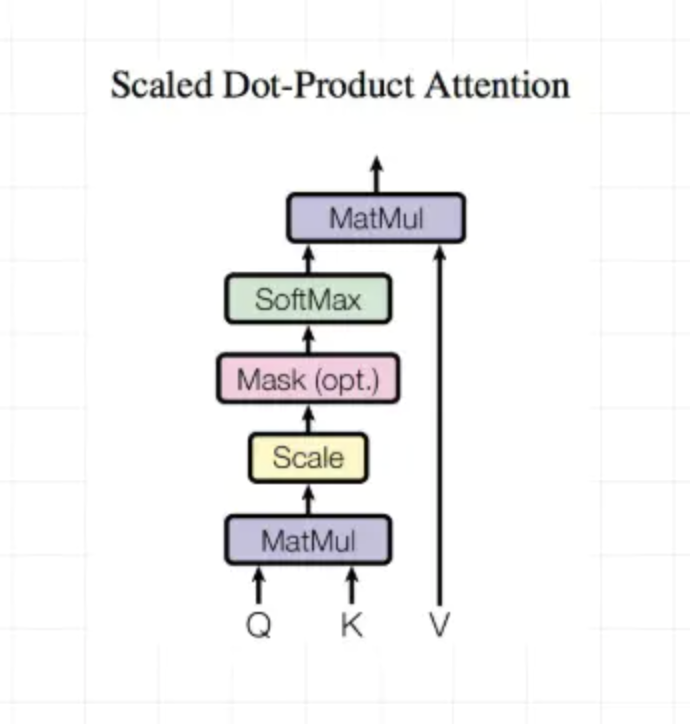Dot-Product计算相似度

outputs = tf.matmul(Q,tf.transpose(K,[0,2,1]))
outputs = outputs / (K.get_shape().as_list()[-1] ** 0.5)


key_masks = tf.sign(tf.abs(tf.reduce_sum(keys,axis=-1)))
paddings = tf.ones_like(outputs) * (-2 ** 32 + 1)


diag_vals = tf.ones_like(outputs[0,:,:])
tril = tf.contrib.linalg.LinearOperatorTriL(diag_vals).to_dense()


# scaled
outputs = tf.nn.softmax(outputs)
# Dropout
outputs = tf.layers.dropout(outputs,rate = dropout_rate,training = tf.convert_to_tensor(is_training))
# Weighted sum
outputs = tf.matmul(outputs,V)
# Residual connection
outputs += queries
# Normalize
outputs = normalize(outputs)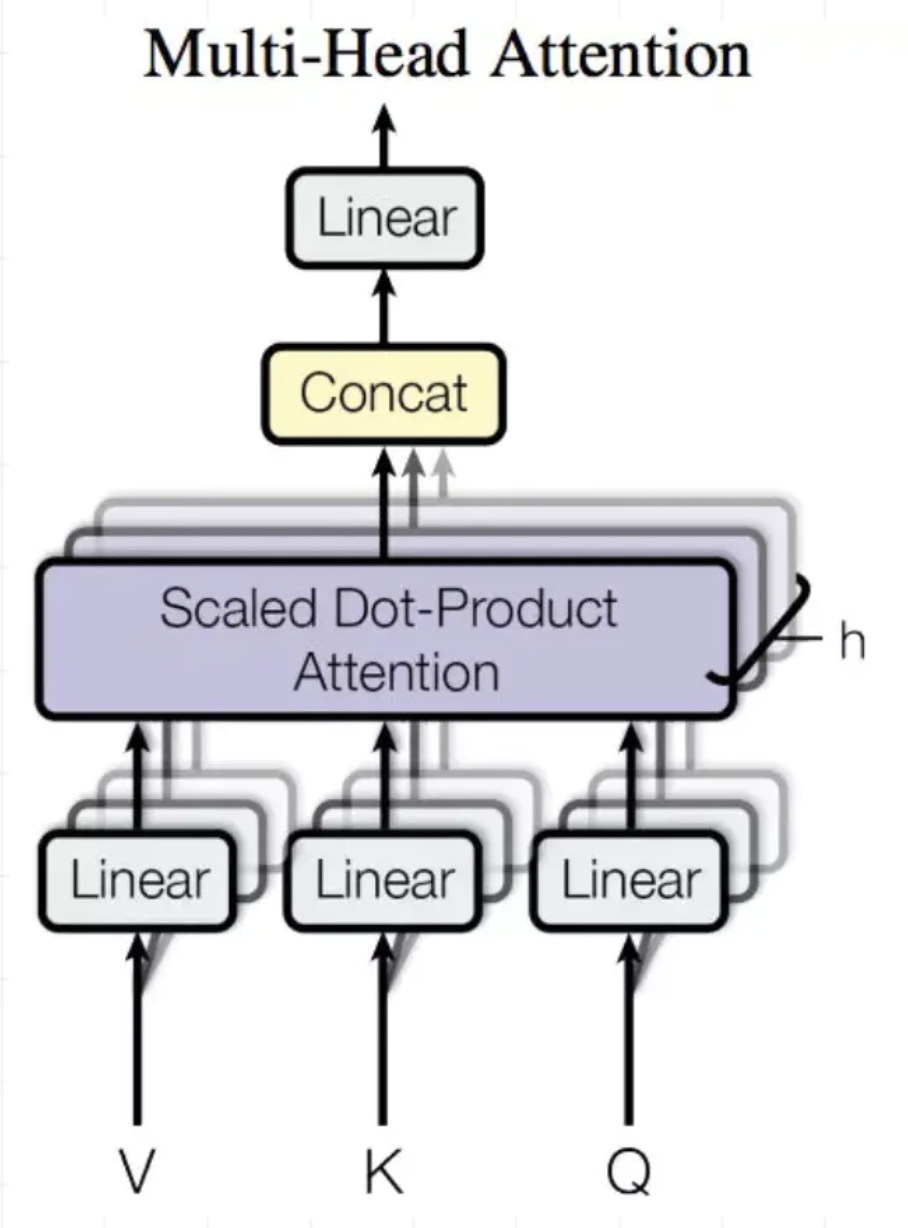## SDM论文笔记

### 回顾与引入(ABSTRACT+1,2两小节)

SDM：SDM: Sequential Deep Matching Model for Online Large-scale Recommender System

1）用户的行为中存在一些误点击行为，通过self-attention来降低这种影响；
2）用户可能对不同物品的关注点不同。

Gate自适应长短期融合的原因：

### 建模&模型架构/结构(第3节)

1）日志中标记了同样的session ID
2）虽然session ID不相同，但是相邻的行为间隔小于10min
3）最长的session长度为50，超过50的划分到前一个session

sdk埋点中session_id定义：应用进入前台生成session_id,退至后台10秒以上重新进入前台重新生成session_id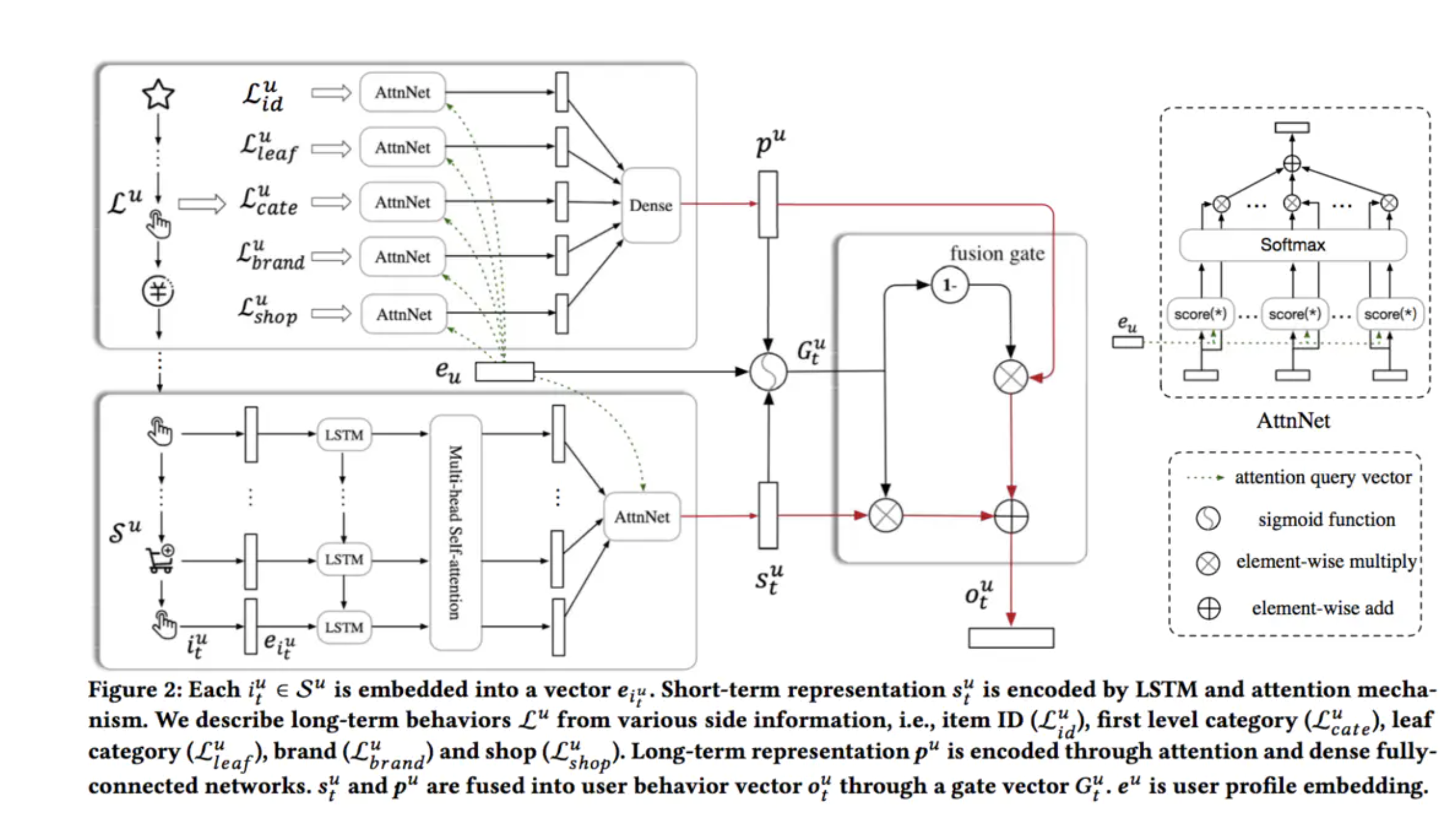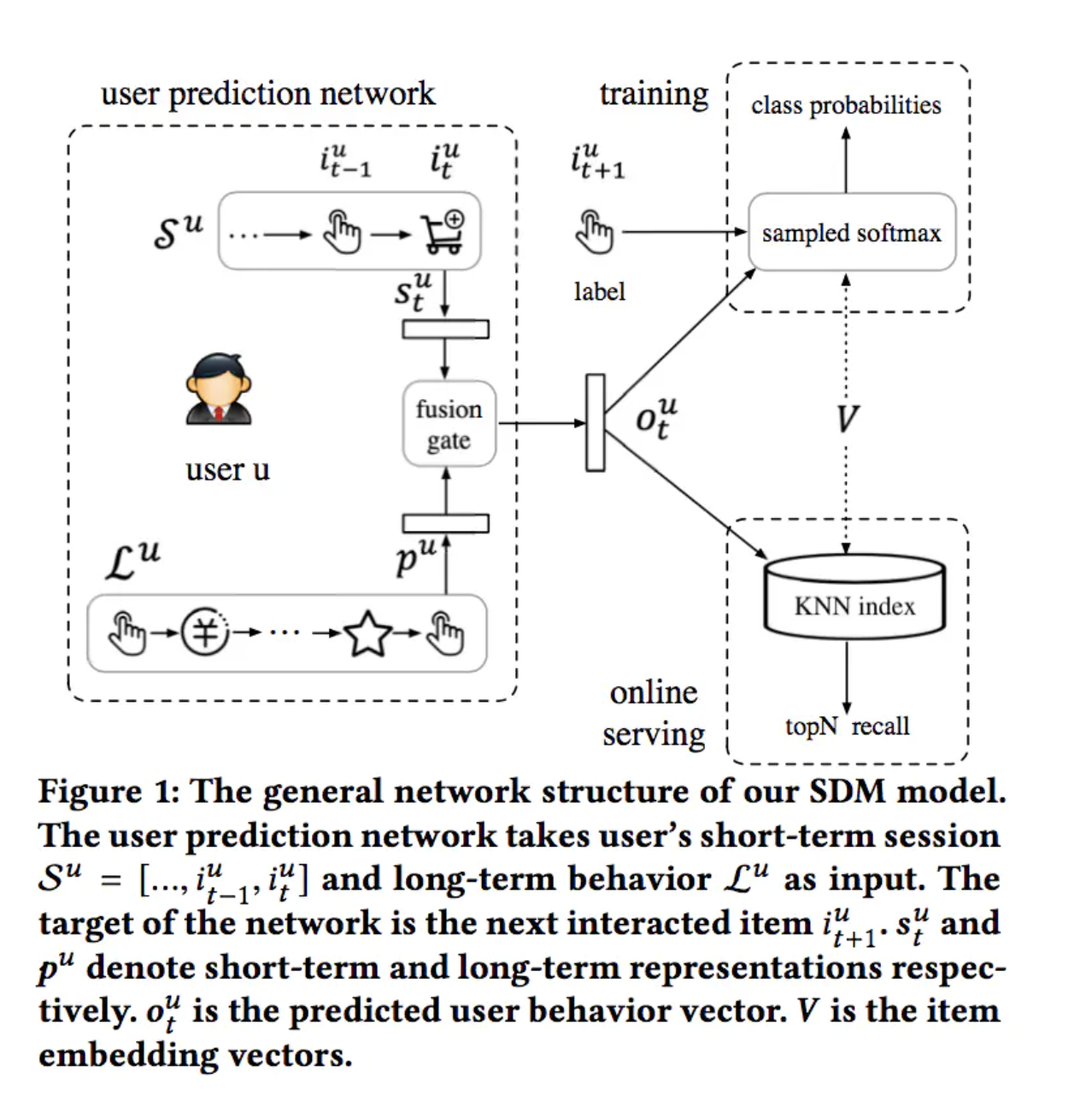User Attention层

$\alpha_{k}=\frac{\exp \left(\hat{h}\_{k}^{u T} e_{u}\right)}{\sum_{k=1}^{t} \exp \left(\hat{h}\_{k}^{u T} e_{u}\right)}$

$s_{t}^{u}=\sum_{k=1}^{t} \alpha_{k} \hat{h}\_{k}^{u}$

$G_{t}^{u}=\operatorname{sigmoid}\left(W^{1} e_{u}+W^{2} s_{t}^{u}+W^{3} p^{u}+b\right)$

$o_t^u=\left(1-G_t^u\right) \odot p^u+G_t^u \odot s_t^u$

### (线上线下)实验设计&实验分析(第4,5,6小节)

1)淘宝数据集：8天内行为物品大于40的活跃用户，但过滤行为超过1000 items的异常用户+过滤出现5次以下的item+过滤长度小于2的session(训练阶段)；前7天做训练第8天测试；长期行为限制为20；
2)京东数据机：3周训练1周测试；其余与淘宝数据机相同；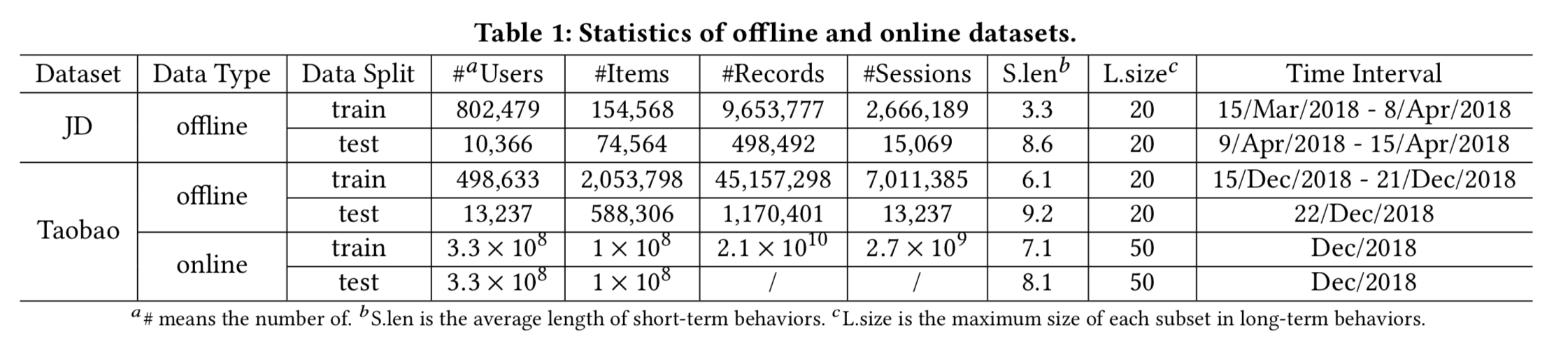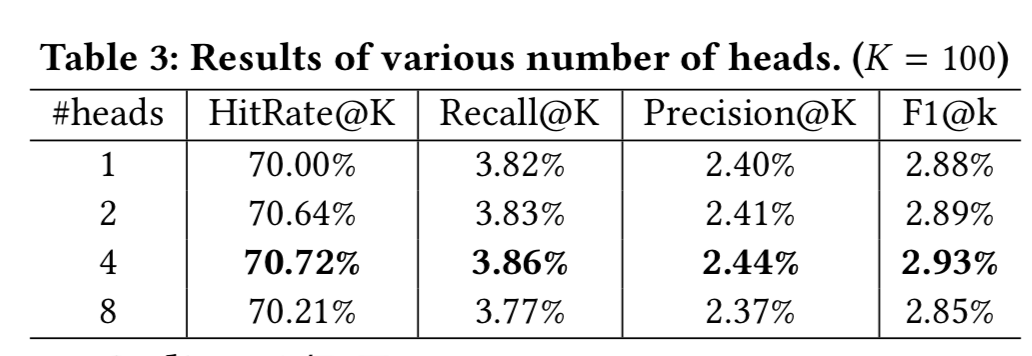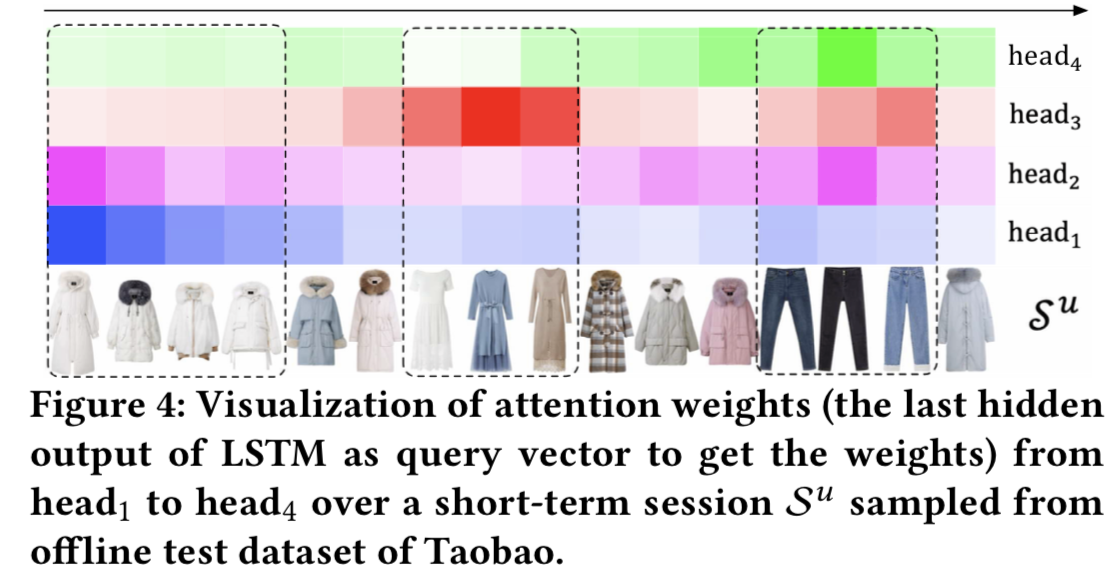2)融合门的实验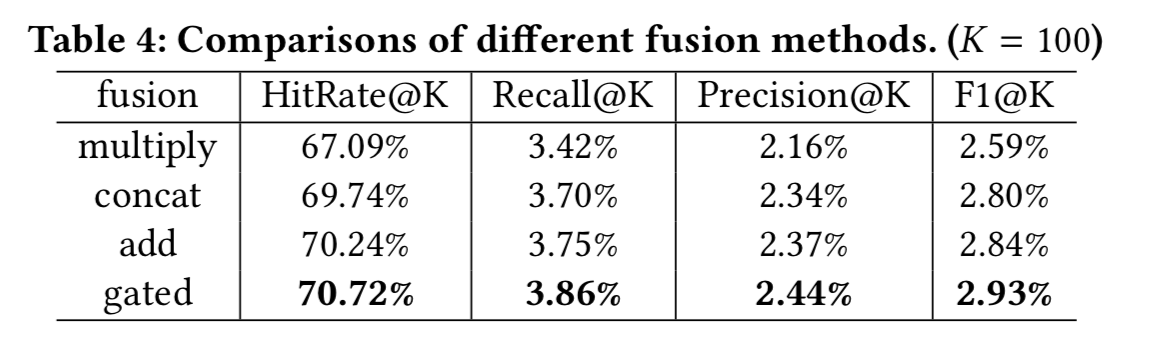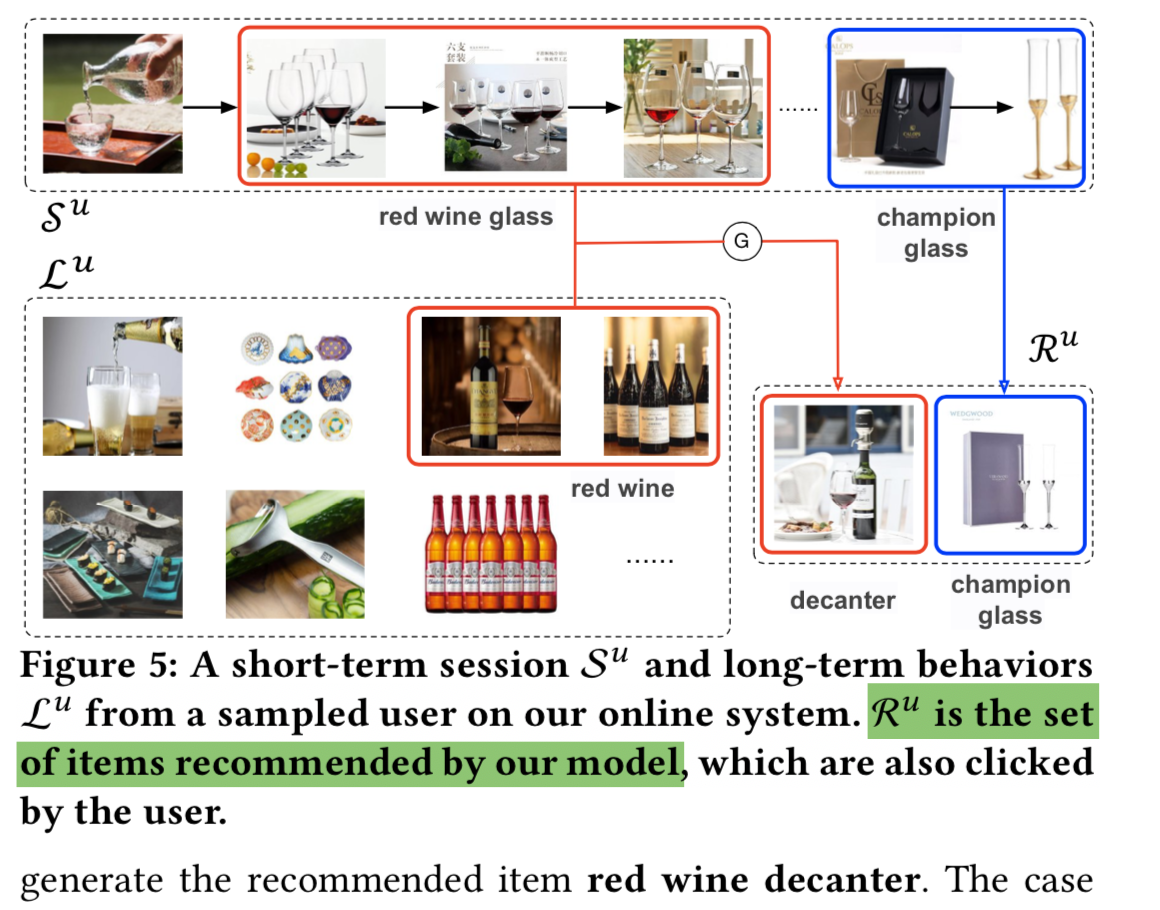# 附录

posted @ 2020-06-29 23:55  混沌战神阿瑞斯  阅读(554)  评论(0编辑  收藏